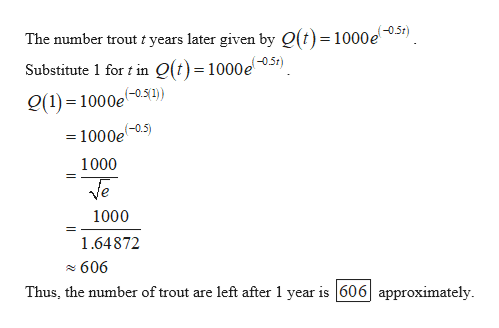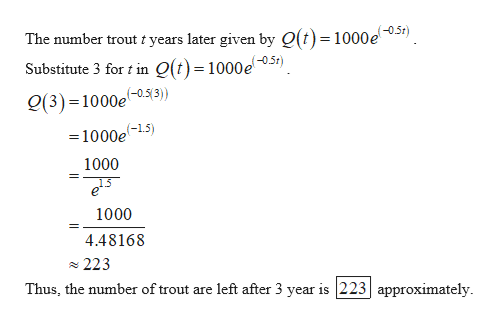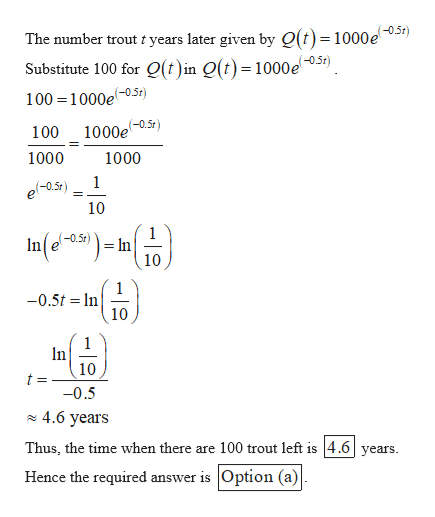# A fishery stocks a pond with 1000 young trout.  The number of trout t years later is given by Q(t) = 1000e(−0.5t).  Graph the number of trout against time.  Do this on extra paper.How many trout are left after 1 year ____________________________?Find Q(3) and interpret your answer in terms of trout _____________________?At what time are there 100 trout left _________________________________?  a.A. Approximately 606 Trout;  B. Approximately 223 and means that after 3 years      the pond will have about 223 trout left.  C.  Approximately 4.6 years b.A. Approximately 606 Trout;  B. Approximately 223 and means that after 3 years      the pond will have about 223 trout left.  C.  Approximately 1.6 years c.A. Approximately 606 Trout;  B. Approximately 2231 and means that after 3 years      the pond will have about 223 trout left.  C.  Approximately 4.6 years d.A. Approximately 900 Trout;  B. Approximately 223 and means that after 3 years      the pond will have about 223 trout left.  C.  Approximately 1.6 years e.None of these

Question
1 views

A fishery stocks a pond with 1000 young trout.  The number of trout t years later is given by Q(t) = 1000e(−0.5t).  Graph the number of trout against time.  Do this on extra paper.

1. How many trout are left after 1 year ____________________________?
2. Find Q(3) and interpret your answer in terms of trout _____________________?
3. At what time are there 100 trout left _________________________________?

 a. A. Approximately 606 Trout;  B. Approximately 223 and means that after 3 years      the pond will have about 223 trout left.  C.  Approximately 4.6 years b. A. Approximately 606 Trout;  B. Approximately 223 and means that after 3 years      the pond will have about 223 trout left.  C.  Approximately 1.6 years c. A. Approximately 606 Trout;  B. Approximately 2231 and means that after 3 years      the pond will have about 223 trout left.  C.  Approximately 4.6 years d. A. Approximately 900 Trout;  B. Approximately 223 and means that after 3 years      the pond will have about 223 trout left.  C.  Approximately 1.6 years e. None of these
check_circle

Step 1

(a)

Find the number of trout are left after 1 year.help_outlineImage TranscriptioncloseThe number trout t years later given by O(t)= 1000e) Substitute 1 for t in Q(t)=1000e0.51) (1) 1000e01) =1000e05) 1000 1000 1.64872 606 Thus, the number of trout are left after 1 year is 606 approximately fullscreen
Step 2

(b)

Find the number of trout are left after 3 years.help_outlineImage TranscriptioncloseThe number trout t years later given by O(t)= 1000e) Substitute 3 for t in Q(t)=1000e0.51) g(3) =1000e-05(3) =1000e-15) 1000 1000 4.48168 223 Thus, the number of trout are left after 3 year is 223 approximately fullscreen
Step 3

(c)

Find the time when there a...help_outlineImage TranscriptioncloseThe number trout t years later given by O(t)= 1000e051) Substitute 100 for Q(t)in Q(t)=1000e 100 1000e0.5t) 100 1000et) 1000 1000 1 (-0.5t 10 In(e-25) (-0.5r) = In 10 1 -0.5t n 10 1 In 10 t -0.5 4.6 years Thus, the time when there are 100 trout left is 4.6years Hence the required answer is Option (a) fullscreen

### Want to see the full answer?

See Solution

#### Want to see this answer and more?

Solutions are written by subject experts who are available 24/7. Questions are typically answered within 1 hour.*

See Solution
*Response times may vary by subject and question.
Tagged in

### Functions